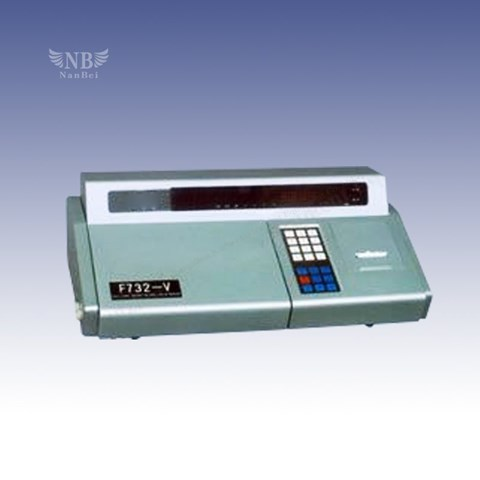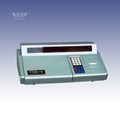Hi, Guest!
• 0
• Nanbei
• NB26-S Double-beam digital display mercury analyzer# NB26-S Double-beam digital display mercury analyzer

Update Terakhir 06 / 12 / 2022
Minimal Pembelian 1 Unit

CALL
Bagikan
DESKRIPSI PRODUK

## Detail NB26-S Double-beam Digital Display Mercury Analyzer

Testing Instrument

product details
NB26-V intelligent mercury analyzer

Product introduction:

The instrument with the computer system, significantly enhanced functionality and can calculate the linear regression equation, standard deviation and coefficient of variation, and in accordance with the parameters of the linear regression equation to calculate the content of the sample, and a print output function.

Indicators:

Sensitivity: not less than 0.05 mg / l

Measuring range: 0-10.0 micrograms / liter (20 mm reduction flask add 5 ml standard sample and 1 ml reducing agent)

Stability: reading drift ≤ ± 2 words / 3 minutes (at A = 0 Office)

Precision: coefficient of variation ≤ 5%

Power supply voltage: 220 volts

Instrument Weight: 12 kg

Dimensions: 510 × 330 × 155 mm3

Features:

Portable smart mercury analyzer greatly improved drift and noise, improved sensitivity and accuracy, and has a direct reading of the concentration of functions and values hold function to make the analysis more convenient to operate.
The instrument with a microcomputer system, features significant enhancements, to calculate the linear regression equation, standard deviation and coefficient of variation, and in accordance with the parameters of the linear regression equation to calculate the content of the sample, and printout.

The advantages of measuring fast, simple operation, digital display, direct reading, is the ideal tool for laboratory measurement of mercury.

Scope:

This instrument is suitable for environmental monitoring, health and epidemic prevention, water, chemical and other industries for the measurement of water, air, soil, food, cosmetics, chemical raw materials, the mercury content.

NB26-S double-beam digital display mercury analyzer

Introduction:

The instrument with the computer system, significantly enhanced functionality and can calculate the linear regression equation, standard deviation and coefficient of variation, and in accordance with the parameters of the linear regression equation to calculate the content of the sample, and a print output function.

Indicators:

Sensitivity: not less than 0.1 micrograms / liter

Measuring range: 0-10.0 micrograms / liter (20 ml flask add 5 ml restore standard sample and 1 ml reducing agent)

Stability: the specified measuring range, reading words drift ≤ ± 2/3 min

Precision: coefficient of variation ≤ 5%

Power supply voltage: 220 volts

Instrument Weight: 12 kg

Dimensions: 510 × 300 × 135 mm3

NB26-G single-beam digital display mercury analyzer

Indicators:

Sensitivity: not less than 0.1 micrograms / liter

Measuring range: 0-10.0 micrograms / liter (20 mm reduction flask add 5 ml standard sample and 1 ml reducing agent)

Stability: the predetermined measuring range, the reading drift ≤ ± 2 words / 1.5 minutes

AC voltage: 220 volts

Instrument Weight: 9 kg

Dimensions: 360 × 310 × 160 mm3